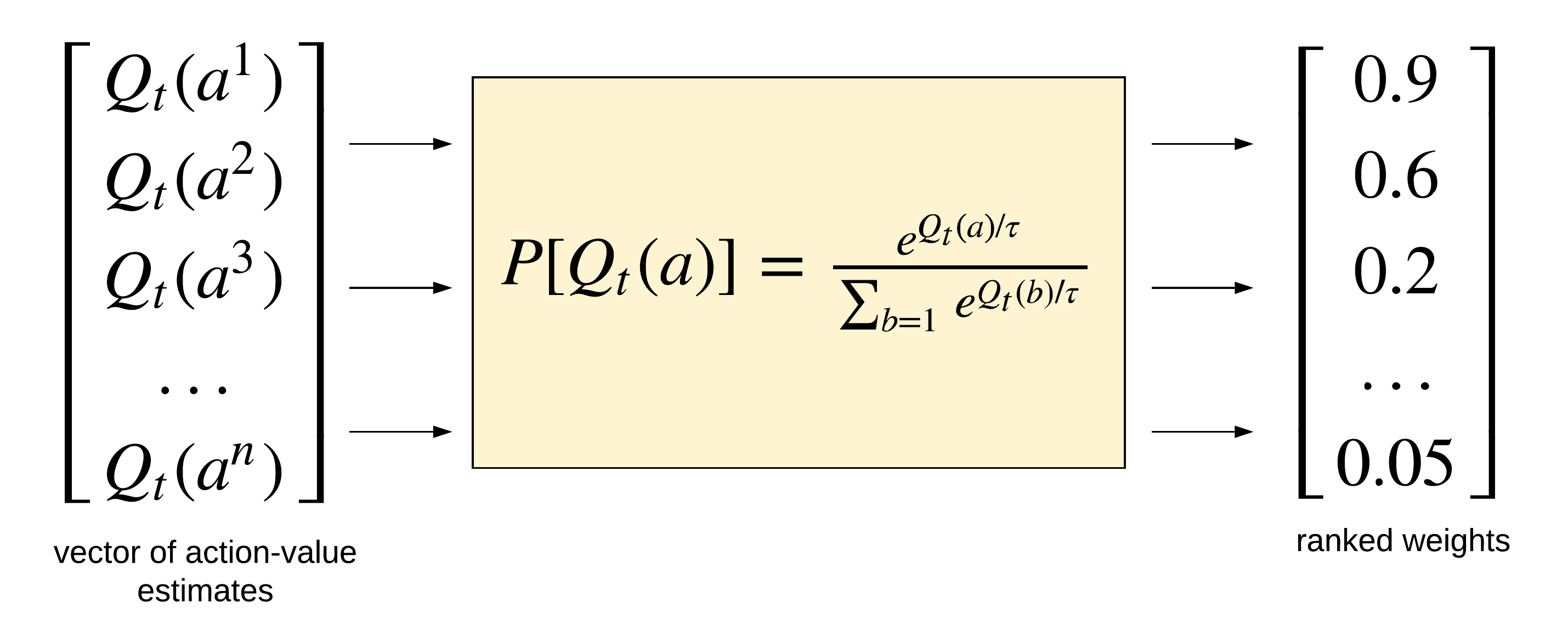The goal of the Reinforcement learning Agent is to maximize long-term rewards or values. To estimate the value of an action in the Agent-Environment feedback framework, we will somehow have to make-use of rewards, which are cheap and are immediately perceived to find expected values which will then be used to optimize action selection. This problem of estimating values to find better actions, intrinsically involves balancing-out exploration and exploitation. This section will evaluate a few approaches to estimating values.

### The sample-average method

The true (or actual) value of an action, denoted as $Q^{*}(a)$, is the ensemble average of rewards received for that action. This true value of an action is unknown by the reinforcement learning Agent. On the other hand, the estimated value of an action, denoted as $Q_{t}(a)$, can be evaluated as the ensemble average of rewards received when action $a$ is selected after $t$ iterations. That is to say, if action $a$ has been selected $n$ times after $t$ iterations, we can write $Q_t(a)$ as:

When expanded, this becomes:

This method of estimating the value of an action is called the sample-average method because as $t \rightarrow \infty$, the estimated value of an action, $Q_t(a)$ approaches the actual value of an action, $Q^{*}(a)$. Having settled on a simple method for estimating the value of an action, we now examine how the Agent may use these estimates to select actions to optimize long-term rewards.

### $\epsilon$-greedy action-value method

An immediately obvious method is for the Agent to always choose the action with the highest value, $a^*$, that is, one for which $Q_t(a^*) = \max_a Q_t(a)$. In doing this, the Agent always exploits its current knowledge in hopes of maximizing its long-term rewards without sampling other actions to perhaps find better alternatives. This greedy behaviour in action selection by the Agent invariably leads to sub-optimal rewards. Without an Agent taking sometime to explore, better actions resulting in long-term rewards will not be discovered.

To mitigate this acute greediness, the Agent can be made to randomly (with uniform distribution) select other actions independently of the action-value estimates with probability, $\epsilon$. The parameter, $\epsilon$, is usually a tiny probability value (e.g. 0.1, 0.001). Near-greedy action selection methods with probability, $\epsilon$ are called $\epsilon$-greedy methods. Over time (i.e. asymptotically) as $t \rightarrow \infty$, by uniformly selecting an action with probability, $\epsilon$, every action will be sampled well-enough for $Q_t(a)$ to converge to $Q^{*}(a)$. The pseudocode for the $\epsilon$-greedy action-value method is shown in Algorithm 1.

### Softmax action selection method

For an Agent to select desirable actions, the $\epsilon$-greedy methods explores the action space with probability, $\epsilon$. When exploring, the Agent selects other actions uniformly or equally. However, by uniformly selecting other actions, the worst or most un-desirable action may be selected and are likely to be detrimental where the penalty is especially high.

The softmax function mitigates this drawback by ranking actions (or assigning weights to actions) by their action-value estimates. The choice of actions influenced by their weights stands to reduce the likelihood of an Agent selecting the worst actions.

The softmax function for finding the probability of choosing an action uses the Boltzmann (or Gibbs) distribution. The Boltzmann distribution in statistical mechanics gives the probability that a system will have a particular state (in this case, the ranked weights) as a function of the energy of the state and the temperature of the system, $\tau$ (see Figure [fig:softmax_function]{reference-type=”ref” reference=”fig:softmax_function”}). Here, the energy of the state is the action-value estimates, $Q_t(a)$. The softmax function is defined as:

where,

• $Q_t(a)$ is the vector of action-value estimates. This vector contains the sample-averages for the finite actions the Agent can make as it interacts with the Environment.

• $\tau$ is the temperature factor. The parameter $\tau$ controls the magnitude of the weight assigned to the action-value estimates. When $tau$ is high, more actions are more likely to be selected by the Agent and as such increases the likelihood of selecting an undesirable action. Conversely, when $\tau$ is low, actions with higher action values estimates and consequently higher weights are more likely to be selected by the Agent. It turns out that when $\tau \rightarrow 0$, the softmax action-selection method acts like a purely greedy action-selection method.Left vector. contains action-value estimates, $Q_t(a)$, derived from the sample-average method. Right vector. contains the ranked weights as a function of the action-value estimates and the temperature parameter, $\tau$.

The Softmax function works by taking the exponent of each action-value estimate and then normalizing them by the sum of the exponents of all the estimated action-values. By normalizing the exponents of the action-values estimates, the softmax function maintains a probability output between 0 and 1.

On Euler’s number, $e$. Euler’s number, $e$ is an important constant in mathematics. The constant $e$ is related to growth functions and rate of change and was discovered by Jacob Bernoulli when studying compound interest. Bernoulli discovered that with continuous and more frequent compounding, a base amount of 1 unit will reach 2.718281828459045.… The number, $e$, is defined as the limit that the compounding interval, $n$ grows, (i.e. $n \rightarrow \infty$). It is expressed as:

Euler then came around and proved that $e$ is an irrational number that equaled:

and can be written as:

In the softmax function, the constant $e$ will convert any negative action-values into $0$ or a positive number. In this way, when we add the sum of the exponents, it will give us a correct normalization.

The code in Listing 1 shows the Python implementation of the softmax method.

Listing 1: Implementation of the Softmax method

The following are the weights of the action-value estimates, $Q_t(a)$, computed by the softmax function.

[0.018232808170459785,
0.6017784080828135,
0.029465574564373497,
0.023489557280065236,
0.01293438520141483,
0.09091175262774233,
0.02859473522943678,
0.08171377709912017,
0.047302159570014206,
0.06557684217455982]


### Bibliography

• Narendra, K. S., & Thathachar, M. A. (2012). Learning automata: An introduction. Courier Corporation.
• Sutton, R. S., & Barto, A. G. (1998). Reinforcement learning: An introduction. MIT press.